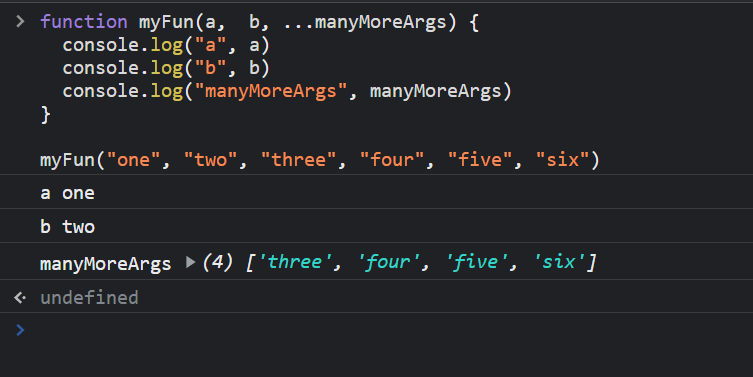GFG App
Open AppBrowser
Continue

# What is spread, default and rest parameters in JavaScript ?

Default Parameter: It is used to give the default values to the arguments, if no parameter is provided in the function call.

Syntax:

```function fnName(param1 = defaultValue1, ..., paramN = defaultValueN) {
...
}```

Example 1: In the below example, the first function gives result 7 whereas the second function call will be “undefined” as we did not pass any second argument.

## Javascript

 ` `

Output:

```7
NaN```

Example 2: In this example, we use default parameters in which we generally give a default value if no argument is provided. We take a default value of “b” so that in the second function call, we are not providing any argument, and its default value is taken.

## Javascript

 ` `

Output:

```7
8```

Spread Operator:  It is another operator provided through ES6 it generally spreads data of array/list.

In the following example, we are calculating min of all the numbers

Example 1:

## Javascript

 ` `

Output:

`-1`

Example 2: Consider that we have an array instead of a list then the above min() function will not work and it will give “NaN”.

## Javascript

 ` `

Output:

`NaN`

Example 3:  When …arr is used, it generally spreads the arr values in the min() function.

## Javascript

 ` `

Output:

`-1`

Rest Operator: It allows a function to accept an indefinite number of arguments if we are not sure how many arguments will receive.

Syntax:

```function f(a, b, ...args) {
...
}```

Example: In the below example, we are using the rest parameter which allows taking indefinite parameters.

## Javascript

 ` `

Output:My Personal Notes arrow_drop_up